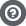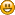Hello Guest

# [Solved] Mandelbrot Set pixelation, failed to solve with BigDecimal.

• 1 Replies
• 2438 Views####Jameth13

•• 5##### [Solved] Mandelbrot Set pixelation, failed to solve with BigDecimal.
« on: March 25, 2014, 14:27:30 »
Hi,

I am trying to render the Mandelbrot Set. I have implemented it successfully with this code using doubles:

Code: [Select]
`public class MandelbrotSetDouble { private int width; private int height; private double x, y, xc, yc, tmp; public MandelbrotSetDouble(int width, int height) { this.width = width; this.height = height; } public ArrayList<Tuple3Int> loop(double zoom, double offSetX, double offSetY, int maxIter) { ArrayList<Tuple3Int> list = new ArrayList<Tuple3Int>(); for (int ix = 0; ix < width; ix++) { for (int iy = 0; iy < height; iy++) { x = y = 0; xc = ((ix - (width / 2)) / zoom) + offSetX; yc = ((iy - (height / 2)) / zoom) + offSetY; for (int iter = 0; iter < maxIter; iter++) { if (((x * x) + (y * y)) < 4) { tmp = x * x - y * y + xc; y = 2.0 * x * y + yc; x = tmp; }else{ list.add(new Tuple3Int(ix, iy, iter)); break; } } } } return list; } }`
Then I converted all the code to using BigDecimal so I could render deeper into the Mandelbrot Set:
Code: [Select]
`public class MandelbrotSetBD { private int width; private int height; private int scale = 32; private int round = 0; private BigDecimal x = new BigDecimal("0").setScale(scale, round); private BigDecimal y = new BigDecimal("0").setScale(scale, round); private BigDecimal xc = new BigDecimal("0").setScale(scale, round); private BigDecimal yc = new BigDecimal("0").setScale(scale, round); private BigDecimal temp1 = new BigDecimal("0").setScale(scale, round); private BigDecimal temp2 = new BigDecimal("0").setScale(scale, round); private BigDecimal four = new BigDecimal("4").setScale(scale, round); private BigDecimal two = new BigDecimal("2").setScale(scale, round); public MandelbrotSetBD(int width, int height) { this.width = width; this.height = height; } public ArrayList<Tuple3Int> loop(String zoom, String offSetX, String offSetY, int maxIter) { System.out.println("Starting generating Mandelbrot Set using BigDecimal with scale=" + scale + "..."); ArrayList<Tuple3Int> list = new ArrayList<Tuple3Int>(); BigDecimal offSetXbd = new BigDecimal(offSetX); BigDecimal offSetYbd = new BigDecimal(offSetY); BigDecimal zoombd = new BigDecimal(zoom); BigDecimal ixbd; BigDecimal iybd; BigDecimal widthbd; BigDecimal heightbd; for (int ix = 0; ix < width; ix++) { for (int iy = 0; iy < height; iy++) { ixbd = new BigDecimal(String.valueOf(ix)); iybd = new BigDecimal(String.valueOf(iy)); widthbd = new BigDecimal(String.valueOf(width)); heightbd = new BigDecimal(String.valueOf(height)); x = new BigDecimal("0").setScale(scale, round); y = new BigDecimal("0").setScale(scale, round); xc = ixbd.subtract(widthbd.divide(two)); xc = xc.multiply(zoombd); xc = xc.add(offSetXbd); yc = iybd.subtract(heightbd.divide(two)); yc = yc.multiply(zoombd); yc = yc.add(offSetYbd); for (int iter = 0; iter < maxIter; iter++) { temp1 = x.multiply(x).setScale(scale, round); temp1 = temp1.add(y.multiply(y)).setScale(scale, round); if (temp1.compareTo(four) == -1) { temp2 = x.multiply(x).setScale(scale, round).subtract(y.multiply(y).setScale(scale, round)).setScale(scale, round).add(xc).setScale(scale, round); y = two.multiply(x).setScale(scale, round).multiply(y).setScale(scale, round).add(yc).setScale(scale, round); x = temp2.setScale(scale, round); }else{ list.add(new Tuple3Int(ix, iy, iter)); break; } } } } System.out.println("Done!"); return list; } }`
With the scale set to 32 it should be twice as accurate as a double but it pixelates at the EXACT same point. Even when I change the scale to anywhere from 16 to 64 it makes NO DIFFERENCE.
I am completely stumped.
I can't run the code without scaling the BigDecimals because it takes far too long to compute the set.

I think I must have a bottleneck somewhere that is stopping me from seeing the benefits from using BigDeciomal but I can't find it.

James

https://mega.co.nz/#!iIVimQaY!KPIMO84fkjWUm8ZLvbNp8lsBd5Mi1B6abo4GKEofooc.
Please let me know what you think so far!
(This version is using doubles.)
« Last Edit: March 25, 2014, 21:18:21 by Jameth13 »####Jameth13

•• 5##### Re: [Help] Mandelbrot Set pixelation, failed to solve with BigDecimal.
« Reply #1 on: March 25, 2014, 21:18:03 »
I honestly have no idea how, but I've fixed it!I was messing around, trying to debug it when it just worked all of a sudden.
I can now render the Mandelbrot Set as deep as I am willing to wait for.